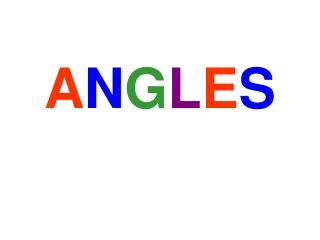DownloadDownload PresentationA N G L E S

# A N G L E S

Download Presentation## A N G L E S

- - - - - - - - - - - - - - - - - - - - - - - - - - - E N D - - - - - - - - - - - - - - - - - - - - - - - - - - -
##### Presentation Transcript

1. ANGLES

2. What is an angle? • Two rays that share the same endpoint form an angle. • The point where the rays intersect is called the vertex of the angle. Vertex Ray Angle

3. Here are some examples of angles.

4. Angle Measurements • We measure the size of an angle using degrees. The symbol for degrees is o • Here are some examples of angles and their degree measurements.

5. Acute Angles • An acute angle is an angle measuring between 0 and 90 degrees. • The following angles are all acute angles.

6. Obtuse Angles • An obtuse angle is an angle measuring between 90 and 180 degrees. • The following angles are all obtuse.

7. Right Angles • A right angle is an angle measuring exactly 90 degrees. • The following angles are both right angles.

8. Straight Angle • A straight angle is exactly 180 degrees.

9. Measuring Angles • You can measure angles using a protractor.

10. Naming Angles < DEF OR < FED The vertex always goes in the middle D • There are 3 ways to name angles: • Using 3 letters • Using 1 letter • Using a number E F <K K <6 6

11. <K OR <JKL OR <LKJ <J OR <MJK OR <KJM J K M L <M OR <LMJ OR <JML <L OR <MLK OR <KLM

12. PRACTICE TIME!

13. AcuteAngle

14. ObtuseAngle

15. RightAngle

16. StraightAngle

17. GREATJOB!!

18. Complementary Angles Two Angles are Complementary if they add up to 90 degrees (a Right Angle).

19. Supplementary Angles Two Angles are Supplementary if they add up to 180 degrees (straight angle).

20. Practice questions out of the textbook: pg. 4181-4, 8-11 OL Pg. 4221-3 (estimate the measure) , 8-14, 20-23, 27-35 PAP Pg. 422 11-33 PAP Pg. 42713-17, 22-24